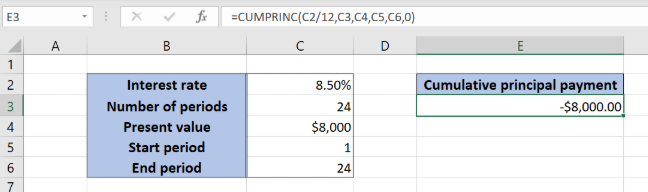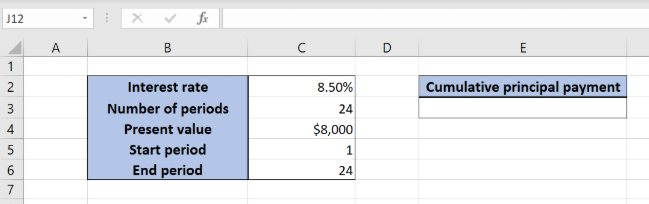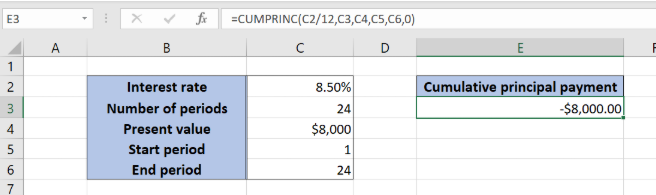Get instant live expert help with Excel or Google Sheets“My Excelchat expert helped me in less than 20 minutes, saving me what would have been 5 hours of work!”

#### Post your problem and you’ll get Expert help in seconds.

Your message must be at least 40 characters
Our professional Expert are available now. Your privacy is guaranteed.

# How to Calculate Cumulative Loan Principal Payments in Excel

Excel allows a user to calculate a cumulative loan principal payment for any period using the CUMPRINC function. This step by step tutorial will assist all levels of Excel users in calculating a cumulative principal payment for a selected period.Figure 1. The result of the function

## Syntax of the CUMPRINC Formula

The generic formula for the CUMPRINC function is:

`=CUMPRINC (rate, nper, pv, start_period, end_period, type)`

The parameters of the CUMPRINC function are:

• rate – an interest rate for the period (the annual rate divided by 12)
• nper – the total number of payments for a loan
• pv – the present value of a loan
• start_period – a start period for calculating a cumulative principal payment
• end_period – an end period for calculating a cumulative principal payment
• type –  specifies when the payments are made: at the end of a period (0) or at the beginning of a period (1).

## Setting up Our Data for the CUMPRINC FunctionFigure 2. Data that we will use in the CUMPRINC example

Let’s look at the structure of the data we will use. In the cell C2, we have the annual interest rate for the loan. In the cell, C3 is the total number of payments, while in the C5 is the present value of the loan. Cells C5 and C6 contain the start and end period. We want to get the result of the CUMPRINC function in the cell E3.

## Calculate the CUMPRINC for the selected period

In our example, we want to get the cumulative principal payment for the whole period of the loan in the cell E3. Because of that the start period is 1, and the end is 24. The interest rate is 8.50%, and the present value of the loan is \$8,000.Figure 3. Using the CUMPRINC function to calculate the cumulative principal payment for the period

The formula looks like:

`=CUMPRINC(C2/12, C3, C4, C5, C6, 0)`

The parameter rate is C2/12, as we must pass the monthly interest rate to the function. The nper is the cell C4 (24), while the start_period is C5 (1) and the end_period is C6 (24). The pv is in the C5 (\$8,000). The type is 0, as the payments are being made at the end of the period.

Finally, the result in the cell E3 is -\$8,000.00, which is the cumulative principal payment for the periods 1-24. The result is always negative, as it is the payment.

To apply the CUMPRINC function, we need to follow these steps:

• Select cell E3 and click on it
• Insert the formula: `=CUMPRINC(C2/12, C3, C4, C5, C6, 0)`
• Press enter

Most of the time, the problem you will need to solve will be more complex than a simple application of a formula or function. If you want to save hours of research and frustration, try our live Excelchat service! Our Excel Experts are available 24/7 to answer any Excel question you may have. We guarantee a connection within 30 seconds and a customized solution within 20 minutes.

### Did this post not answer your question? Get a solution from connecting with the expert.Another blog reader asked this question today on Excelchat:## Subscribe to Excelchat.coAnother blog reader asked this question today on Excelchat: